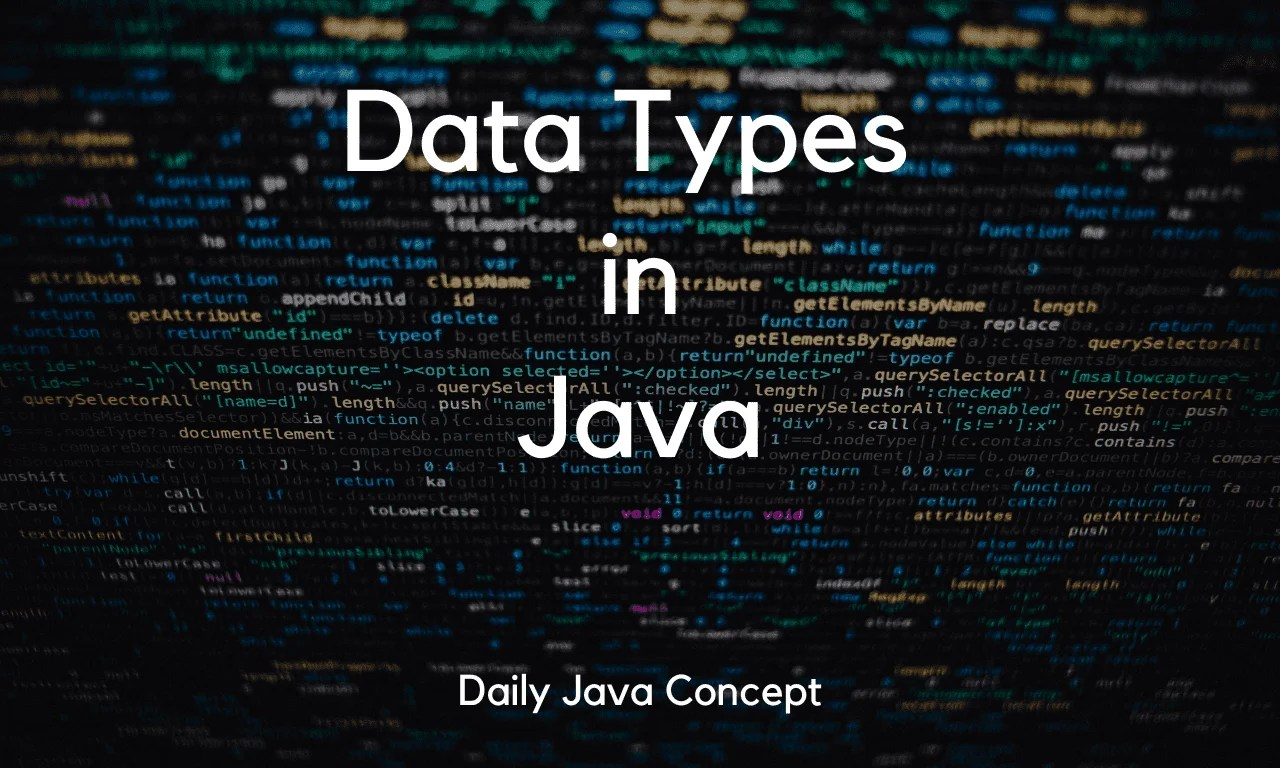# Data Types in Java

0
128Java is a strongly typed programming language, which means that every variable and expression in Java has a data type. The data type determines the values that a variable can store and the operations that can be performed on that variable. In this blog, we will explore the different data types available in Java.

## Primitive Data Types

Java has eight primitive data types, including boolean, char, byte, short, int, long, float, and double. These are the building blocks of Java data types.

Syntex for any primitive data type is shown below:

<datatype_name> <variable_name> = <value_assignment>;

Syntax
``````//for example, declaring int data type:
int age = 12;``````

The primitive data types in Java are:

### byte

The byte data type in Java is an 8-bit signed two’s complement integer. It has a minimum value of -128 and a maximum value of 127. The byte data type is used when you need to save memory in large arrays, as it takes up less space than other data types such as int or long.

Syntax
``````byte age = 12;
// In variable age, value 12 assigned. ``````

### short

short is a primitive data type that is used to represent a 16-bit signed two’s complement integer. It has a minimum value of -32,768 and a maximum value of 32,767. Example:

Syntax
``````short sold = 200;
//In variable sold, value 200 assigned.``````

### int

It is a 32-bit signed integer type. The range of values that can be stored in an int variable is -2,147,483,648 (-2^31) to 2,147,483,647 (2^31 – 1). Example:

Syntax
``````int numberOfProductSold = 300;
// In variable numberOfProductSold, value 300 assigned.``````

### long

It is a 64-bit signed integer type. The range of values that can be stored in a long variable is -9,223,372,036,854,775,808 to 9,223,372,036,854,775,807. Example:

Syntax
``````long lotSize = 400L;
//In Variable lotSize, value 400 assigned. L represents Long Datatype value.``````

### float

It is a 32-bit floating-point type. Example:

Syntax
``````float marks = 10.0f;
// In variable marks, 10.0 float value assigned with decimal point. f in the end denotes float datatype value.``````

### double

It is a 64-bit floating-point type. Example:

JavaScript
``````double d = 20.0;
// In variable d, 20.0 assigned.``````

### boolean

It can store either true or false values. Example:

JavaScript
``````boolean isActive = true;
// In boolean variable isActive, boolean value true assigned.``````

### char

It is a 16-bit Unicode character type. It has a minimum value of `'\u0000'` (or 0) and a maximum value of `'\uffff'` (or 65,535 inclusive). value assigned to char variable should be in the single quotes (‘). Example:

JavaScript
``````char ch = 'A';
// In char variable ch, value 'A' assigned.``````

## Reference Data Types

Reference data types are also known as object references, and they are used to store references to objects. Java has several reference data types, including arrays, strings, and class types. The reference data types in Java are:

### Object

It is the base class for all classes in Java. An Object in Java is an instance of a class. It is a basic building block of Java programming language. An object is a real-world entity that can be represented in computer memory and can be manipulated by the program.

Syntax
``Object obj = new Object();``

### String

A string in Java is a sequence of characters. It can be used to store and manipulate text data. In Java, strings are objects of the java.lang.String class. The value assigned to the String Type variable should be in the double quotes (” “). Example:

Syntax
``````String str = "Hello, World!";
// In String variable str, value "Hello, World!" assigned.``````

### Array

An array is a data structure that stores a fixed number of values of the same data type. If you wanted to learn more about arrays: Arrays in Java

Syntax
``````int[] numbers = {1, 2, 3, 4, 5};
// Any integer type array defined with array values with length of 5.``````

### Class

A class is a blueprint for creating objects, providing initial values for state (member variables or instance variables) and implementations of behavior (member functions or methods).

Java – Employee Class
``````public class Employee {
private String name;
private int age;
public Employee(String name, int age) {
this.name = name;
this.age = age;
}
public String getName() {
return name;
}
public int getAge() {
return age;
}
}``````

## Default Values

In conclusion, data types play a crucial role in Java programming and are used to store and manipulate values. Understanding the different data types available in Java and their uses will help you become a more effective and efficient Java programmer.

Latest Posts: